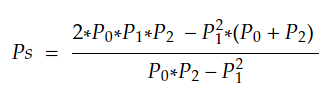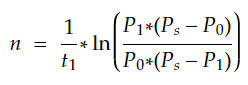top of page
Search
• APSEd

# Logistic Curve Method of Population Forecasting with Solved Example

The logistic curve method of population forecasting is a method to predict the population using the logistic curve of population growth. The concept of logistic curve and formulas to predict the population as per the logistic curve method are discussed further.

## What is a Logistic Curve

In an ideal environment, populations grow at an exponential rate. The growth curve of these populations is smooth and becomes increases steeply over time. However, exponential growth is not possible because of factors such as limitations in food, competition for other resources, disease etc. Populations eventually reach the carrying capacity or saturation capacity of the environment, causing the growth rate to slow nearly to zero. This produces an S-shaped curve of population growth known as the logistic curve.Logistic curve of population growth

## Logistic Curve Method

As discussed, this method uses the logistic curve of population growth. Therefore, it uses the equation of the logistic curve to directly predict the population. Equation of the logistic curve is given as,

P = Ps/(1+(m*ln^-1(n*t))), pictorial representation of the same is shown below,Logistic curve equation

where,

m = (Ps - Po)/Po,

Ps - saturation population given by, Ps = (2*Po*P1*P2 - P1^2(Po+P2))/(Po*P2 - P1^2),

pictorial representation of the same is shown below,Saturation population formula

n = (1/t1)*ln((Po*(Ps-P1))/(P1*(Ps-Po)), pictorial representation of the same is shown below,n formula in logistic curve equation

Po - population at to years,

P1 - population at t1 years,

P2 - population at t2 years,

t1 - number of years between to and t1,

t2 - number of years between to and t2,

t - number of years between 'to' and required year,

t2 = 2*t1 (in general).

## Solved Example

Question: Using the data given below find the population for the year 2021.

 Year Population 1991 80000 2001 250000 2011 480000

Solution:

Step 1: Given data,

Po = 80,000 at to = 0 years,

P1 = 250,000 at t1 = 10 years,

P2 = 480,000 at t2 = 20 years,

Step 2: Find saturation population Ps using the formula Ps = (2*Po*P1*P2 - P1^2(Po+P2))/(Po*P2 - P1^2),

Ps = 655,602

Step 3: Find the value of m using the formula m = (Ps - Po)/Po,

m = (655602 - 80000)/80000

m = 7.195

Step 4: Find the value of using the formula n = (1/t1)*ln((Po*(Ps-P1))/(P1*(Ps-Po)),

n = -0.1489

Step 5: Find the population for the required year using the formula P = Ps/(1+(m*ln^-1(n*t))),

t = 30 (number of years between to and t)

P = 655602/(1 + (7.195*ln^-1(-0.1489*30)))

P = 6,05,436 is the population for the year 2021.

## Practise Problem

For more insights and to know more about other population forecasting methods refer to the video lecture below.

Want to be a part of the APSEd community? Fill out the form below and we will get back to you!